Physics
Easy

Question

# Richa is sitting in a merry-go-round.  The time period of circular motion if it forms a circle of 56 m radius. The speed of rotation is 40 m/s.

## 2.8 pi s280 pi s0.14 pi s28 pi sHint:

## The correct answer is: 2.8 pi s

Physics

### A girl is sitting in a merry-go-round.  The time period of circular motion of the merry-go-round is 8 sec and it forms a circle of 40 m radius. What is the speed of rotation?

1. 30.9 m/s
2. 810.4 m/s
3. 480.34 m/s
4. 31.4 m/s

### A girl is sitting in a merry-go-round.  The time period of circular motion of the merry-go-round is 8 sec and it forms a circle of 40 m radius. What is the speed of rotation?

1. 30.9 m/s
2. 810.4 m/s
3. 480.34 m/s
4. 31.4 m/s
Physics

1. Up
2. Down
3. Left
4. Right

1. Up
2. Down
3. Left
4. Right
Physics

1. Up
2. Down
3. Left
4. Right

### What is the direction of the velocity at point mentioned if the ball is revolving anticlockwise?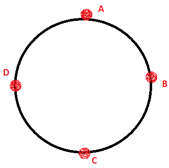At point C

1. Up
2. Down
3. Left
4. RightPhysics

1. Up
2. Down
3. Left
4. Right

1. Up
2. Down
3. Left
4. Right
Physics

1. Up
2. Down
3. Left
4. Right

1. Up
2. Down
3. Left
4. Right
Physics

### As the velocity of the object moving in a uniform circular motion increases, the time period _______.

1. Increases
2. Decreases
3. Increases exponentially
4. Decreases exponentially

### As the velocity of the object moving in a uniform circular motion increases, the time period _______.

1. Increases
2. Decreases
3. Increases exponentially
4. Decreases exponentiallyPhysics

### The time taken for one complete circle is called the _____.

1. Time period
2. Frequency
3. Angular velocity
4. Velocity

### The time taken for one complete circle is called the _____.

1. Time period
2. Frequency
3. Angular velocity
4. Velocity
Physics

### An object is moving at a uniform speed in a counterclockwise, horizontal circle. Which of the following images correctly depicts the force and velocity at a point in time?

1.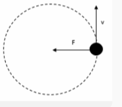2.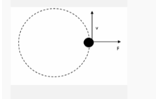3.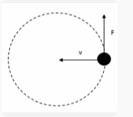4.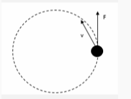### An object is moving at a uniform speed in a counterclockwise, horizontal circle. Which of the following images correctly depicts the force and velocity at a point in time?

1.2.3.4.Physics

1. 29.43 m
2. 20.24 m
3. 30.48 m
4. 45.97 m

### A boy moves a tied stone above his head in circles, time period of circular motion is 4.4 sec, and its speed of rotation is 42 m/s. What is the radius of the circle formed?

1. 29.43 m
2. 20.24 m
3. 30.48 m
4. 45.97 mPhysics

### A boy moves a tied stone above his head in circles, what is the time period of circular motion if it forms a circle of 20 cm radius. The speed of rotation is 23 m/s.

1. 0.25 s
2. Sqrt (0.05) s
3. 0.05 s
4. 0.1 s

### A boy moves a tied stone above his head in circles, what is the time period of circular motion if it forms a circle of 20 cm radius. The speed of rotation is 23 m/s.

1. 0.25 s
2. Sqrt (0.05) s
3. 0.05 s
4. 0.1 s
Physics

1. 0.5 m/s
2. 0.6 m/s
3. 0.7 m/s
4. 0.8 m/s

1. 0.5 m/s
2. 0.6 m/s
3. 0.7 m/s
4. 0.8 m/s
Physics

1. Up
2. Down
3. Left
4. Right

### What is the direction of the velocity at point mentioned if the ball is revolving clockwise.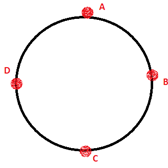At point D

1. Up
2. Down
3. Left
4. RightPhysics

1. Up
2. Down
3. Left
4. Right

1. Up
2. Down
3. Left
4. Right
Physics

1. Up
2. Down
3. Left
4. Right

1. Up
2. Down
3. Left
4. Right
Physics

1. Up
2. Down
3. Left
4. Right

### What is the direction of the velocity at point mentioned if the ball is revolving clockwise.At point A

1. Up
2. Down
3. Left
4. Right#### With Turito Foundation.#### Get an Expert Advice From Turito.